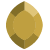# What is the formule for calculating the power spectrum of profile based data?Posts: 1i am trying to calculate depth to basement by using 1D fft, on this Power spectrum equation is slope divided by 4pi. it is correct or not for calculating slope of the energy spectrum.